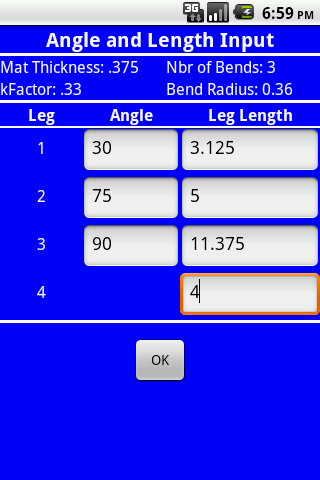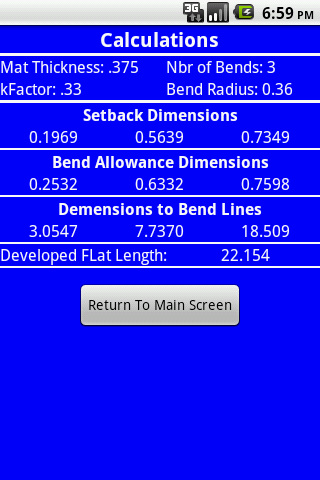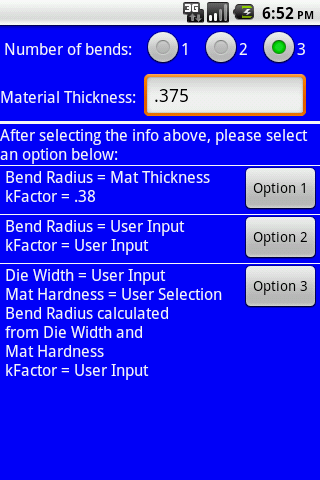# Bend Line CalculatorBend Line Calculator is designed to calculate a number of important features when bending sheet metal or flat bar material. The bending operation assumes a V-die in an air bending situation.

This program allows up to 3 bends with a maximum bend angle of 90 degrees for each bend. The calculations for each bend are:

Setback distance

Bend allowance

Distance from part end to each bend line

Total developed flat length